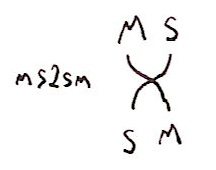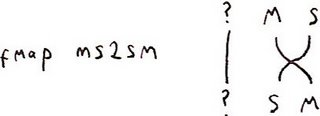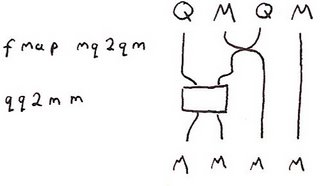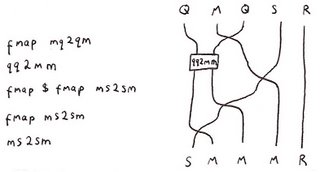## Friday, September 08, 2006

### More Low Cost Geometric Algebra

In my second last post I showed how large algebraic structures could be built out of smaller ones using parametric polymorphism. In fact, for certain types, the type constructor acts like a tensor product in the category of algebras. It turns out that many of these structures are isomorphic to each other and Haskell provides a nice way to talk about these isomorphisms.

Before that I should say a bit more about Clifford algebras. These are an important tool in many branches of mathematics - algebraic topology, K-theory, representation theory and in theoretical physics. But one interesting aspect is that they can provide an alternative to the usual vector language of geometry. Many people will be familiar with the use of quaternions to represent 3D rotations. Clifford algebras generalise this to N-dimensions.

Before proceeding, note the footnote below on notation. And rather than write literate Haskell I've put the code here.

Back on topic: the first isomorphisms I should mention are

A⊗B≅B⊗A

for any A and B. For example HR(2)≅R(2)⊗H. As Haskell types this says that Quaternion (Matrix a) is isomorphic to Matrix (Quaternion a). Or in English: the 2×2 matrices of quaternions are isomorphic to the quaternions whose components are 2×2 matrices. This isn't completely trivial. For example let ms2sm be the isomorphism
`ms2sm :: (Num a) => Matrix (Split a) -> Split (Matrix a)ms2sm (M (S a b) (S c d) (S f g) (S h j)) =    S (M a c f h) (M b d g j)`

You can see that it's a little like a matrix transpose operation.

We can draw this pictorially as:Note that the same function, because it is polymorphic, also gives an isomorphism Matrix (Split (Quaternion a)) -> Split (Matrix (Quaternion a)). But what about the isomorphism Quaternion (Matrix (Split a)) -> Quaternion (Split (Matrix a))? We can get this almost for free using the functoriality of the constructors. The isomorphism is fmap ms2sm. We can illustrate this as:If we implement each of the pairwise flips then in combination with fmap we can get any permutation of the constructors. And you can probably imagine some pretty braid-like diagrams describing the process. (I could mutter something about symmetric monoidal categories here.)

These isomorphisms are almost trivial - they just swap elements around. But pick up any book that discusses Clifford algebras and you'll find a bunch of extra isomorphisms listed:

CCCC

CHC(2)

HHR(4)

For example, a quaternion of quaternions is much the same as a 4×4 matrix. We choose to represent the latter as a 2×2 matrix of 2×2 entries with the isomorphism
`qq2mm :: (Num a) => Quaternion (Quaternion a) -> Matrix (Matrix a)qq2mm (Q (Q a b c d) (Q f g h j) (Q k l m n) (Q p q r s)) =    M (M (a+g+m+s) (b-f-n+r)  (f-b-n+r)  (a+g-m-s))      (M (c+j-k-q) (d-h+l-p)  (h-d+l-p)  (c+j+k+q))      (M (j-c+k-q) (d+h+l+p)  (-d-h+l+p) (j-c-k+q)      (M (a-g+m-s) (-b-f+n+r) (b+f+n+r)  (a-g-m+s))`

(That took me a few minutes of actual work to unpack the proof of that in Spin Geometry by Lawson and Michelson so it's no longer for free!) There's a really important reason why you might want to do this. It takes 16 multiplications of the underlying elements to multiply two quaternions but it only takes 8 multiplications to multiply 2×2 matrices. A Matrix (Matrix a) can therefore be multiplied four times as fast as a Quaternion (Quaternion a). So being able to switch back and forth between repesentations is useful.

I think I now have a simple kit of parts for manipulating Clifford algebras efficiently. If I have time I should try to write a complete library for N-dimensional Clifford algebras. I wonder if I could apply it to path counting methods?

Back to isomorphisms. I want to mention two in particular. One is

Cn+8=R(16)⊗Cn

The actual isomorphism is
`bott :: Num a => Quaternion (Matrix (Quaternion (Matrix a))) -> Matrix (Matrix (Matrix (Matrix a)))bott = qq2mm . fmap mq2qm`

It's prettier viewed pictorially:As I've mentioned before, this isomorphism is an example of Bott periodicity. It pops up in many places in mathematics, for example in Supergravity or K-Theory.

This is an interesting isomorphism:Remember that Split a is essentially a pair of objects of type a. So we can define
`cliff72left,cliff72right :: Cliff7 -> Matrix (Matrix (Matrix R))cliff72left = first . ms2sm . fmap ms2sm . (fmap (fmap ms2sm)) . qq2mm . fmap mq2qmcliff72right = second . ms2sm . fmap ms2sm . (fmap (fmap ms2sm)) . qq2mm . fmap mq2qm`

This gives not one, but two different ways to represent an element of C7 as an 8×8 matrix. But there's more to it than that. The main real-world application for quaternions is for describing rotations - they're well used in the 3D graphics world for example. Elements of C7 can be used to describe rotations in 8D. (Modulo a sign ambiguity that should be familiar to anyone who's played with quaternions.) So what he have is that a rotation in 8D can be represented with 3 different 8×8 matrices: the usual one, and the two coming from C7 (modulo the sign). This has many implications in physics. For example these three matrices describe how bosons, left-handed fermions and right-handed fermions transform under rotations. In 8-dimensions these matrices are all 8×8 making 8D a special dimension for doing physics. By a roundabout path this is closely linked to the 10D of superstring theory. This "theewayness" is known as triality which John Baez has written about a few times.

I'm getting to like this type constructor as tensor product viewpoint. I can see a lot of mathematics that be expressed nicely in it. General relativity and knot theory are two that come to mind...

I go back and forth between conventional mathematical notation and Haskell. I also have one letter abbreviations I use in function names and diagrams. The translation table is:

 Mathematics Haskell Abbreviation R⊕R Split s C Complex c H Quaternion q R(2) Matrix m Cn CliffN C'n CliffN'

Labels: ,bluetechnx said...

Hi,

I am *not* a Math major...and retook Calculs3 like 4 times. BUT, one thing I have learned is that problems can be viewed in many lights. pictures, mathematical notation, English or Code.

For those who haven't even taken calculs, diffyQ's or linear algebra I think that your blog posts are very readable, engaining and a joy to read. Because, of the many facets by which you demonstrate a problem and its solutions.

I may have a hard time at mathematics, but having useful insights and discourses by people such as yourself make it a joy to read/study on *further* and try to get better at!

thank you,
David G.

Sunday, 10 September, 2006augustss said...sigfpe said...